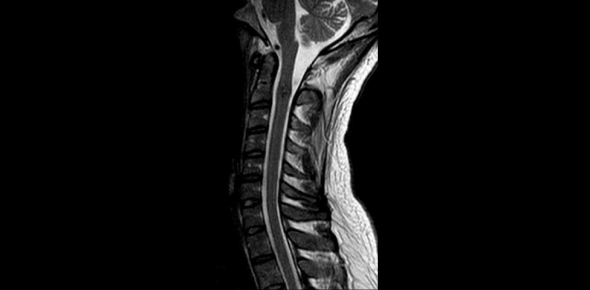# MRI Of The Spinal Column Quiz!

16 Questions | Total Attempts: 170Settings.

• 1.
Refer to the figure below of a sagittal, T2-weighted MRI of the lumbar vertebra. Which arrow points to the superior articular process?
• A.

A

• B.

B

• C.

C

• D.

D

• 2.
Refer to the figure below of a 3D CT of the lumbar spine, lateral view. Which arrow points to the sacral promontory?
• A.

A

• B.

B

• C.

C

• D.

D

• 3.
Refer to the figure below of a 3D CT of the lumbar spine, lateral view. Which arrow points to the body of L3?
• A.

A

• B.

B

• C.

C

• D.

D

• 4.
The most inferior portion of the spinal cord, located at approximately the level of the T12-L1, is called the:
• A.

Cauda equina

• B.

Filum terminale

• C.

Sacral plexus

• D.

Conus medullaris

• 5.
Refer to the figure below. Which arrow points to brachial plexus?
• A.

A

• B.

B

• C.

C

• D.

D

• 6.
Refer to the figure below of an axial, T2-weighted MRI of the lumbar spine with intervertebral disk and zygapophyseal joints. Which arrow points to the nucleus pulposus?
• A.

A

• B.

B

• C.

C

• D.

D

• 7.
Refer to the figure below. Which arrow points to the spinal cord?
• A.

A

• B.

B

• C.

C

• D.

D

• 8.
The brachial plexus arises from which ventral rami?
• A.

C4-C7

• B.

C5-C8 and T1

• C.

C3-C7 and T1

• D.

C5-C7 and T1-T3

• 9.
Refer to the figure below of a sagittal, T2-weighted MRI of the lumbar vertebra. Which arrow points to the inferior articular process?
• A.

A

• B.

B

• C.

C

• D.

D

• 10.
Refer to the figure below of an axial, T2-weighted MRI of the lumbar vertebra. Which arrow points to the cauda equina?
• A.

A

• B.

B

• C.

C

• D.

D

• 11.
Refer to the figure below of a 3D CT of the cervical vertebrae, anterior view. Which arrow points to the transverse foramen of C1?
• A.

A

• B.

B

• C.

C

• D.

D

• 12.
Refer to the figure below. Which arrow points to the brachial plexus?
• A.

A

• B.

B

• C.

C

• D.

D

• 13.
Refer to the figure below. Which arrow points to the middle scalene muscle?
• A.

A

• B.

B

• C.

C

• D.

D

• 14.
Refer to the figure below. Which arrow points to the pituitary gland?
• A.

A

• B.

B

• C.

C

• D.

D

• 15.
Refer to the figure below of a sagittal, T2-wighted MRI of the lumbar vertebra. Which arrow points to the intervertebral foramen?
• A.

A

• B.

B

• C.

C

• D.

D

• 16.
Refer to the figure below of a coronal, T2-weighted MRI of the brain. Which arrow points to the third ventricle?
• A.

A

• B.

B

• C.

C

• D.

D

Related TopicsBack to top